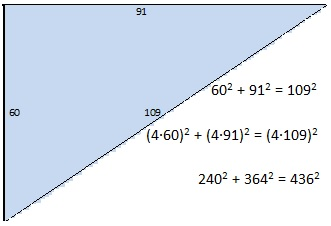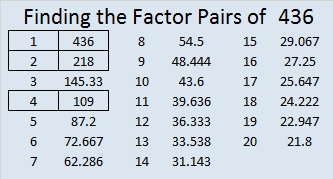# 436 Pythagorean Triples and Level 3

Probably the most famous Pythagorean triple is 3-4-5. It doesn’t matter if the 3-4-5 refers to millimeters, centimeters, inches, meters, or even miles, this relationship will always hold: 3^2 + 4^2 = 5^2.

Likewise, the relationship will still hold if we multiply each number 3-4-5 by the same number. Let’s multiply each of them by 109 to get 327-436-545. Go ahead and test it: 327^2 + 436^2 = 545^2.

The following graphic shows a Pythagorean triple with numbers much larger than the familiar 3-4-5. Still everything is relative: 60-91-109 in millimeters is much smaller than 3-4-5 in miles.You may have noticed that 436 appeared in a couple of those triples I listed above. That’s because 436 = 4 x 109. Some of those side lengths may have seemed large, but try imagining this Pythagorean triple: 436-23760-23764 or this even larger primitive triple: 436-47523-47525. In each of those monstrous cases, the sum of the squares of the first two numbers equals the square of the last number, too!

This puzzle is relatively easy to solve:Print the puzzles or type the factors on this excel file: 12 Factors 2015-03-23

• 436 is a composite number.
• Prime factorization: 436 = 2 x 2 x 109, which can be written 436 = (2^2) x 109
• The exponents in the prime factorization are 2 and 1. Adding one to each and multiplying we get (2 + 1)(1 + 1) = 3 x 2  = 6. Therefore 436 has exactly 6 factors.
• Factors of 436: 1, 2, 4, 109, 218, 436
• Factor pairs: 436 = 1 x 436, 2 x 218, or 4 x 109
• Taking the factor pair with the largest square number factor, we get √436 = (√4)(√109) = 2√109 ≈ 20.8806A Logical Approach to solve a FIND THE FACTORS puzzle: Find the column or row with two clues and find their common factor. Write the corresponding factors in the factor column (1st column) and factor row (top row).  Because this is a level three puzzle, you have now written a factor at the top of the factor column. Continue to work from the top of the factor column to the bottom, finding factors and filling in the factor column and the factor row one cell at a time as you go.## One thought on “436 Pythagorean Triples and Level 3”

1.mathtuition88

Very nice post! I’m really fascinated by Pythagorean Triples.
I wrote a post on using Rational Circles to generate Pythagorean Triples at: http://mathtuition88.com/2014/08/26/rational-parametrization-of-a-circle-pythagorean-triples/